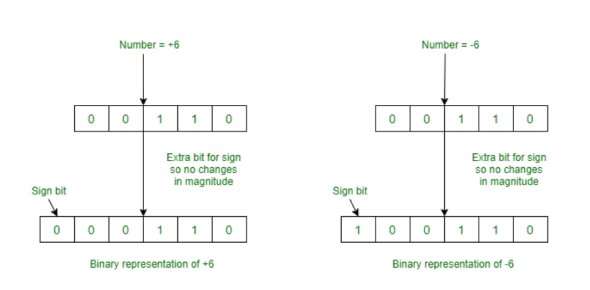# Binary Sytem- Representing Data Quiz

30 Questions | Total Attempts: 88SettingsWhat do you know about the binary system? And how do we represent data using it? You might have learned the basics in your senior high school. And if you have studied computer science well or electronics, you might know the advanced level too. Here are thirty questions

• 1.
Base 2 number system used by computers. Uses 1s and 0s:
• A.

Binary

• B.

Denary

• C.

• 2.
Base 16 number system. Uses digits 0-9 and A-F:
• A.

Binary

• B.

Denary

• C.

• 3.
Base 10 number system. The system we use to count:
• A.

Binary

• B.

Denary

• C.

• 4.
How many bits are there in 1 nibble?
• A.

2

• B.

4

• C.

6

• D.

8

• 5.
How many bits are there in 1 byte?
• A.

2

• B.

4

• C.

6

• D.

8

• 6.
How many bytes are there in 1 megabyte? [Blank]
• 7.
What is the 8 bit binary representation for 134? [Blank]
• 8.
What is the denary representation for binary 01001111? [Blank]
• 9.
What is the 8 bit binary representation for hexadecimal A3? [Blank]
• 10.
Convert the denary number 167 into hexadecimal: [Blank]
• 11.
Convert the hexadecimal number D7 into denary: [Blank]
• 12.
Add the binary numbers 00111111 and 01001100: [Blank] Give your answer as an 8 bit binary number.
• 13.
Add the binary numbers 10111011 and 00101101: [Blank] Give your answer as an 8 bit binary number.
• 14.
Strings are formed on a computer by joining bytes that represent different letters together. Name the character encoding standard that enables up to 128 different commonly used characters, numbers and symbols to be used: [Blank]
• 15.
Using the ASCII table to work out the following word: 01101000     01100101     01101101     01100101     01101110 [Blank]
• 16.
True or false? ASCII has a limited character set of 128 character.
• A.

True

• B.

False

• 17.
True or false? ASCII accommodates all language character sets.
• A.

True

• B.

False

• 18.
True or false? Unicode is a alternative to ASCII and has 4 billion possible characters.
• A.

True

• B.

False

• 19.
The picture shows a black and white image. Assuming that black is stored as 1 and white is stored as 0, express the image as a binary string (2 bytes), which is how it would be represented on a computer: [Blank] Write your answer as 2 bytes separated with a single space, e.g: 01010101 01010101
• 20.
What is the term given to a single dot of colour in a picture?
• A.

Dot

• B.

Spot

• C.

Pixel

• D.

Bit

• 21.
A sound card in a computer works by converting analogue sound to what?[Blank]
• 22.
Identify the units usually referred to when recording sound:
• A.

Bytes

• B.

Kilobytes

• C.

Hertz

• D.

Kilohertz

• 23.
True or false? Lossy and Lossless are the two main methods used for file compression.
• A.

True

• B.

False

• 24.
Select all the reasons for using compression on a computer:
• A.

• B.

To reduce storage space required

• C.

To make it easier to find files

• D.

To make it quicker to save files

• 25.
Select all of the disadvantages to choosing lossy compression over lossless when compressing an image file:
• A.

The image file cannot be restored to original.

• B.

The quality of the image will be reduced

• C.Back to top